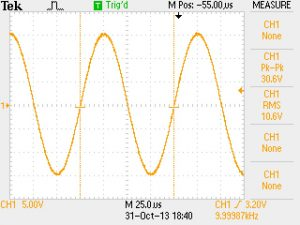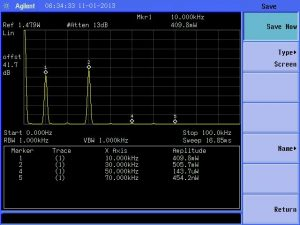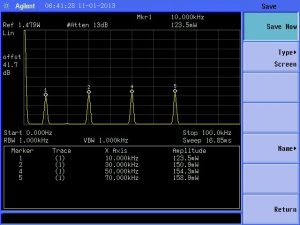# Does Running Multiple Frequencies ‘Reduce’ Power?

When waveforms are generated with multiple frequencies, the power content of each frequency is reduced. The greater the number of frequencies, the greater the power reduction to the point where each power can become insignificant. Using only a few frequencies is more beneficial- after all, that is how most communication equipment operates. However, when many frequencies are added together, each is diluted too greatly to be of any use.  This means that for a given power, more frequencies result in less power per frequency than the one.

Formula to calculate each frequency power: X watts of frequency1 has twice as much power of X watts of frequency1 added to frequency2 (X watts = X/2 watts frequency1 + X/2 watts of frequency2).

Those who run multiple frequencies simultaneously are seeking the benefits of the time savings to use multiple frequencies. BCX Ultra manufacturer decided to cap at 3 simultaneous frequencies (frequency, carrier wave, gating).  Other manufacturers have designed their unit to run multiple frequencies. The reason we do not is the greater the number of frequencies, the greater the power reduction to the point where each power can become insignificant.  In turn the user then has to repeat the frequencies to get the needed dose and dealing with kill off you want the power and the surges (based on wave type). Other manufacturers state differently. We believe running 1 carrier wave & 1 frequency with the most power output for that accessory is the best method for treatment. Having 1 carrier wave carrying multiple frequencies is not as effective. Now they state that if there is enough power delivered so then each frequency will be effective and all is needed is .20 watts. However we limit our metal electrode power output to .75 watts (w 30-35 volts) to stay within safe limits since electricity is the means of delivery thru metal electrodes (this doesn’t apply to the plasma accessories). Most of our clients can’t even handle the full .75 watts (then turn down the power) so we wonder how can someone handle the full power of 2.7watts? Keep in mind our BCX Ultra has a built in 18 watt amplifier so we easily can produce the power. Note our BCX Ultra unit has different accessories that add additional power to go from 20 watts all the way up to 150 watts depending on the accessory.

Below is a 10KHz sinewave with a peak to peak amplitude of 30 volts. The spectrum analyzer shows the power into a fixed impedance load is 1 watt.Below is 10KHz and 30KHz with the same peak to peak amplitude of 30 volts. The power level of the 10KHz frequency has been reduced to 0.4 watts, and the power level of the 30KHz frequency is 0.5 watts.Below is 10, 30, 50, and 70KHz with the same peak to peak amplitude of 30 volts. The power level of the 10KHz frequency has been reduced to 0.12 watts, and the power level of the 30, 50, and 70KHz frequency is about 0.15 watts each.Question: What happened to the rest of the 1 watt of power? It is contained in increasing numbers of harmonic frequencies with very low insignificant power levels. As more frequencies are added together, the waveform approaches a square wave. Mathematically, a square wave is an infinite number of odd harmonic frequencies added together.